## Section 1.3: Functions and Function Notation

### Learning Outcomes

• Determine whether a relation represents a function.
• Find the value of a function.
• Determine whether a function is one-to-one.
• Use the vertical line test to identify functions.
• Graph the functions listed in the library of functions.

A jetliner changes altitude as its distance from the starting point of a flight increases. The weight of a growing child increases with time. In each case, one quantity depends on another. There is a relationship between the two quantities that we can describe, analyze, and use to make predictions. In this section, we will analyze such relationships.

## Determining Whether a Relation Represents a Function

A relation is a set of ordered pairs. The set of the first components of each ordered pair is called the domain and the set of the second components of each ordered pair is called the range. Consider the following set of ordered pairs. The first numbers in each pair are the first five natural numbers. The second number in each pair is twice that of the first.

$\begin{gather}\left\{\left(1,2\right),\left(2,4\right),\left(3,6\right),\left(4,8\right),\left(5,10\right)\right\}\end{gather}$

The domain is $\left\{1,2,3,4,5\right\}$. The range is $\left\{2,4,6,8,10\right\}$.

Note that each value in the domain is also known as an input value. The input values are values of the independent variable which often labeled with the lowercase letter $x$. Each value in the range is also known as an output value. The output values are values of the dependent variable which is often labeled lowercase letter $y$.

A function $f$ is a relation that assigns a single value in the range to each value in the domain. In other words no x-values are repeated. For our example that relates the first five natural numbers to numbers double their values, this relation is a function because each element in the domain, $\left\{1,2,3,4,5\right\}$, is paired with exactly one element in the range, $\left\{2,4,6,8,10\right\}$.

Now let’s consider the set of ordered pairs that relates the terms “even” and “odd” to the first five natural numbers. It would appear as

$\begin{gather}\left\{\left(\text{odd},1\right),\left(\text{even},2\right),\left(\text{odd},3\right),\left(\text{even},4\right),\left(\text{odd},5\right)\right\}\\{ }\end{gather}$

Notice that each element in the domain, $\left\{\text{even,}\text{odd}\right\}$ is not paired with exactly one element in the range, $\left\{1,2,3,4,5\right\}$. For example, the term “odd” corresponds to three values from the domain, $\left\{1,3,5\right\}$ and the term “even” corresponds to two values from the range, $\left\{2,4\right\}$. This violates the definition of a function, so this relation is not a function.

Figure 1 compares relations that are functions and not functions.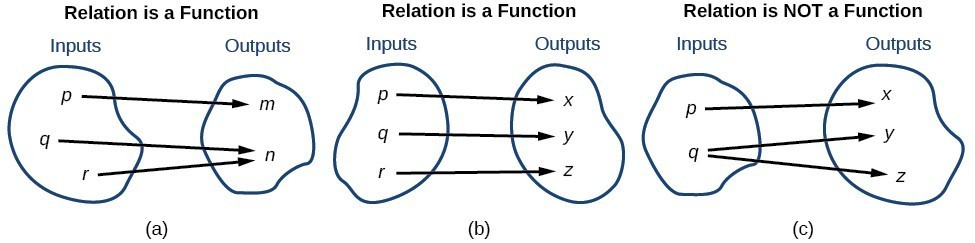Figure 1. (a) This relationship is a function because each input is associated with a single output. Note that input $q$ and $r$ both give output $n$. (b) This relationship is also a function. In this case, each input is associated with a single output. (c) This relationship is not a function because input $q$ is associated with two different outputs.

### A General Note: Function

A function is a relation in which each possible input value leads to exactly one output value. We say “the output is a function of the input.”

The input values make up the domain, and the output values make up the range.

### How To: Given a relationship between two quantities, determine whether the relationship is a function.

1. Identify the input values.
2. Identify the output values.
3. If each input value leads to only one output value, the relationship is a function. If any input value leads to two or more outputs, the relationship as a function.

### Example 1: Determining If Menu Price Lists Are Functions

The coffee shop menu, shown in Figure 2 consists of items and their prices.

1. Is price a function of the item?
2. Is the item a function of the price?

### Example 2: Determining If Class Grade Rules Are Functions

In a particular math class, the overall percent grade corresponds to a grade point average. Is grade point average a function of the percent grade? Is the percent grade a function of the grade point average? The table below shows a possible rule for assigning grade points.

 Percent Grade Grade Point Average 0–56 57–61 62–66 67–71 72–77 78–86 87–91 92–100 0.0 1.0 1.5 2.0 2.5 3.0 3.5 4.0

### Try It

The table below lists the five greatest baseball players of all time in order of rank.

Player Rank
Babe Ruth 1
Willie Mays 2
Ty Cobb 3
Walter Johnson 4
Hank Aaron 5
a) Is the rank a function of the player name?
b) Is the player name a function of the rank?

## Using Function Notation

Once we determine that a relationship is a function, we need to display and define the functional relationships so that we can understand and use them, and sometimes also so that we can program them into computers. There are various ways of representing functions. A standard function notation is one representation that facilitates working with functions.

To represent “height is a function of age,” we start by identifying the descriptive variables $h$ for height and $a$ for age. The letters $f,g$, and $h$ are often used to represent functions just as we use $x,y$, and $z$ to represent numbers and $A,B$, and $C$ to represent sets.

\begin{gathered}\begin{cases}\begin{align}&h\text{ is }f\text{ of }a && \text{We name the function }f;\text{ height is a function of age}. \\ &h=f\left(a\right) && \text{We use parentheses to indicate the function input}\text{. } \\ &f\left(a\right) && \text{We name the function }f;\text{ the expression is read as }f\text{ of }a\text{.''} \end{align} \end{cases}\end{gathered}

Remember, we can use any letter to name the function; the notation $h\left(a\right)$ shows us that $h$ depends on $a$. The value $a$ must be put into the function $h$ to get a result. The parentheses indicate that age is input into the function; they do not indicate multiplication.

We can also give an algebraic expression as the input to a function. For example $f\left(a+b\right)$ means “first add a and b, and the result is the input for the function f.” The operations must be performed in this order to obtain the correct result.

### A General Note: Function Notation

The notation $y=f\left(x\right)$ defines a function named $f$. This is read as $y$ is a function of $x.''$ The letter $x$ represents the input value, or independent variable. The letter $y$, or $f\left(x\right)$, represents the output value, or dependent variable.

### Example 3: Using Function Notation for Days in a Month

Use function notation to represent a function whose input is the name of a month and output is the number of days in that month.

### Example 4: Interpreting Function Notation

A function $N=f\left(y\right)$ gives the number of police officers, $N$, in a town in year $y$. What does $f\left(2005\right)=300$ represent?

### Q & A

Instead of a notation such as $y=f\left(x\right)$, could we use the same symbol for the output as for the function, such as $y=y\left(x\right)$, meaning “y is a function of x?”

Yes, this is often done, especially in applied subjects that use higher math, such as physics and engineering. However, in exploring math itself we like to maintain a distinction between a function such as $f$, which is a rule or procedure, and the output $y$ we get by applying $f$ to a particular input $x$. This is why we usually use notation such as $y=f\left(x\right),P=W\left(d\right)$, and so on.

## Representing Functions Using Tables

A common method of representing functions is in the form of a table. The table rows or columns display the corresponding input and output values. In some cases, these values represent all we know about the relationship; other times, the table provides a few select examples from a more complete relationship.

The table below lists the input number of each month (January = 1, February = 2, and so on) and the output value of the number of days in that month. This information represents all we know about the months and days for a given year (that is not a leap year). Note that, in this table, we define a days-in-a-month function $f$ where $D=f\left(m\right)$ identifies months by an integer rather than by name.

 Month number, $m$ (input) 1 2 3 4 5 6 7 8 9 10 11 12 Days in month, $D$ (output) 31 28 31 30 31 30 31 31 30 31 30 31

The table below defines a function $Q=g\left(n\right)$. Remember, this notation tells us that $g$ is the name of the function that takes the input $n$ and gives the output $Q$.

 $n$ 1 2 3 4 5 $Q$ 8 6 7 6 8

The table below displays the age of children in years and their corresponding heights. This table displays just some of the data available for the heights and ages of children. We can see right away that this table does not represent a function because the same input value, 5 years, has two different output values, 40 in. and 42 in.

 Age in years, $\text{ }a\text{ }$ (input) 5 5 6 7 8 9 10 Height in inches, $\text{ }h\text{ }$ (output) 40 42 44 47 50 52 54

### How To: Given a table of input and output values, determine whether the table represents a function.

1. Identify the input and output values.
2. Check to see if each input value is paired with only one output value. If so, the table represents a function.

### Example 5: Identifying Tables that Represent Functions

Which table, a), b), or c), represents a function (if any)?

a)

Table A
Input Output
2 1
5 3
8 6

b)

Table B
Input Output
–3 5
0 1
4 5

c)

Table C
Input Output
1 0
5 2
5 4

When we know an input value and want to determine the corresponding output value for a function, we evaluate the function. Evaluating will always produce one result because each input value of a function corresponds to exactly one output value.

When we know an output value and want to determine the input values that would produce that output value, we set the output equal to the function’s formula and solve for the input. Solving can produce more than one solution because different input values can produce the same output value.

## Evaluating Functions in Algebraic Forms

When we have a function in formula form, it is usually a simple matter to evaluate the function. For example the function $f\left(x\right)=5 - 3{x}^{2}$ can be evaluated by squaring the input value, multiplying by 3, and then subtracting the product from 5.

### How To: Given the formula for a function, evaluate.

1. Replace the input variable in the formula with the value provided.
2. Calculate the result.

### Example 6: Evaluating Functions

Given the function $h\left(p\right)={p}^{2}+2p$, evaluate $h\left(4\right)$.

### Example 7: Evaluating Functions at Specific Values

Evaluate $f\left(x\right)={x}^{2}+3x - 4$ at

1. $2$
2. $a$
3. $a+h$
4. $\frac{f\left(a+h\right)-f\left(a\right)}{h}$

### Try It

Given the function $g\left(m\right)=\sqrt{m - 4}$, evaluate $g\left(5\right)$.

### Example 8: Solving Functions

Given the function $h\left(p\right)={p}^{2}+2p$, solve for $h\left(p\right)=3$.

### Try It

Given the function $g\left(m\right)=\sqrt{m - 4}$, solve $g\left(m\right)=2$.

## Evaluating Functions Expressed in Formulas

Some functions are defined by mathematical rules or procedures expressed in equation form. If it is possible to express the function output with a formula involving the input quantity, then we can define a function in algebraic form. For example, the equation $2n+6p=12$ expresses a functional relationship between $n$ and $p$. We can rewrite it to decide if $p$ is a function of $n$.

### How To: Given a function in equation form, write its algebraic formula.

1. Solve the equation to isolate the output variable on one side of the equal sign, with the other side as an expression that involves only the input variable.
2. Use all the usual algebraic methods for solving equations, such as adding or subtracting the same quantity to or from both sides, or multiplying or dividing both sides of the equation by the same quantity.

### Example 9: Finding an Equation of a Function

Express the relationship $2n+6p=12$ as a function $p=f\left(n\right)$, if possible.

### Example 10: Expressing the Equation of a Circle as a Function

Does the equation ${x}^{2}+{y}^{2}=1$ represent a function with $x$ as input and $y$ as output? If so, express the relationship as a function $y=f\left(x\right)$.

### Try It

If $x - 8{y}^{3}=0$, express $y$ as a function of $x$.

### Q & A

Are there relationships expressed by an equation that do represent a function but which still cannot be represented by an algebraic formula?

Yes, this can happen. For example, given the equation $x=y+{2}^{y}$, if we want to express $y$ as a function of $x$, there is no simple algebraic formula involving only $x$ that equals $y$. However, each $x$ does determine a unique value for $y$, and there are mathematical procedures by which $y$ can be found to any desired accuracy. In this case we say that the equation gives an implicit (implied) rule for $y$ as a function of $x$, even though the formula cannot be written explicitly.

## Evaluating a Function Given in Tabular Form

As we saw above, we can represent functions in tables. Conversely, we can use information in tables to write functions, and we can evaluate functions using the tables. For example, how well do our pets recall the fond memories we share with them? There is an urban legend that a goldfish has a memory of 3 seconds, but this is just a myth. Goldfish can remember up to 3 months, while the beta fish has a memory of up to 5 months. And while a puppy’s memory span is no longer than 30 seconds, the adult dog can remember for 5 minutes. This is meager compared to a cat, whose memory span lasts for 16 hours.

The function that relates the type of pet to the duration of its memory span is more easily visualized with the use of a table. See the table below.

Pet Memory span in hours
Puppy 0.008
Adult dog 0.083
Cat 16
Goldfish 2160
Beta fish 3600

At times, evaluating a function in table form may be more useful than using equations. Here let us call the function $P$.

The domain of the function is the type of pet and the range is a real number representing the number of hours the pet’s memory span lasts. We can evaluate the function $P$ at the input value of “goldfish.” We would write $P\left(\text{goldfish}\right)=2160$. Notice that, to evaluate the function in table form, we identify the input value and the corresponding output value from the pertinent row of the table. The tabular form for function $P$ seems ideally suited to this function, more so than writing it in paragraph or function form.

### How To: Given a function represented by a table, identify specific output and input values.

1. Find the given input in the row (or column) of input values.
2. Identify the corresponding output value paired with that input value.
3. Find the given output values in the row (or column) of output values, noting every time that output value appears.
4. Identify the input value(s) corresponding to the given output value.

### Example 11: Evaluating and Solving a Tabular Function

Using the table below,

1. Evaluate $g\left(3\right)$.
2. Solve $g\left(n\right)=6$.
 n 1 2 3 4 5 g(n) 8 6 7 6 8

### Try It

Using the table in Example 11, evaluate $g\left(1\right)$ .

## Finding Function Values from a Graph

Evaluating a function using a graph also requires finding the corresponding output value for a given input value, only in this case, we find the output value by looking at the graph. Solving a function equation using a graph requires finding all instances of the given output value on the graph and observing the corresponding input value(s).

### Example 12: Reading Function Values from a Graph

Given the graph in Figure 6,

1. Evaluate $f\left(2\right)$.
2. Solve $f\left(x\right)=4$.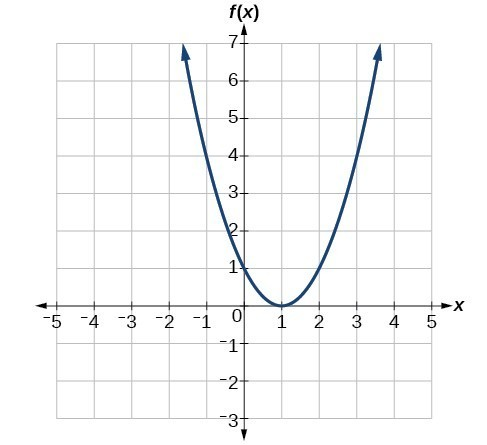Figure 6

### Try It

Using Figure 6, solve $f\left(x\right)=1$.

### Using the Vertical Line Test

As we have seen in some examples above, we can represent a function using a graph. Graphs display a great many input-output pairs in a small space. The visual information they provide often makes relationships easier to understand. By convention, graphs are typically constructed with the input values along the horizontal axis and the output values along the vertical axis.

The most common graphs name the input value $x$ and the output value $y$, and we say $y$ is a function of $x$, or $y=f\left(x\right)$ when the function is named $f$. The graph of the function is the set of all points $\left(x,y\right)$ in the plane that satisfies the equation $y=f\left(x\right)$. If the function is defined for only a few input values, then the graph of the function is only a few points, where the x-coordinate of each point is an input value and the y-coordinate of each point is the corresponding output value. For example, the black dots on the graph in Figure 11 tell us that $f\left(0\right)=2$ and $f\left(6\right)=1$. However, the set of all points $\left(x,y\right)$ satisfying $y=f\left(x\right)$ is a curve. The curve shown includes $\left(0,2\right)$ and $\left(6,1\right)$ because the curve passes through those points.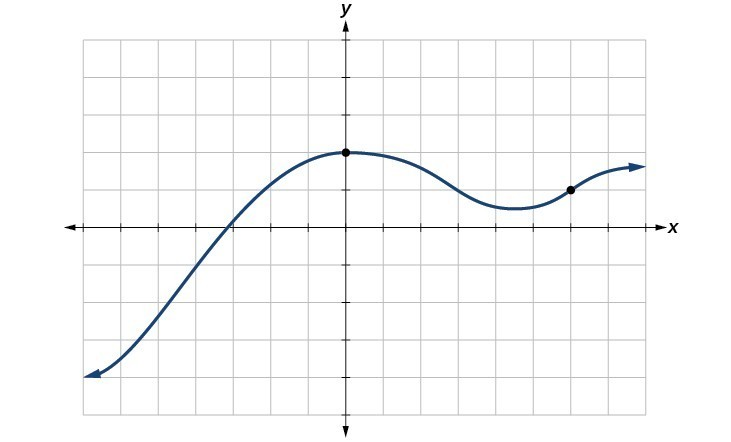Figure 11

The vertical line test can be used to determine whether a graph represents a function. If we can draw any vertical line that intersects a graph more than once, then the graph does not define a function because a function has only one output value for each input value.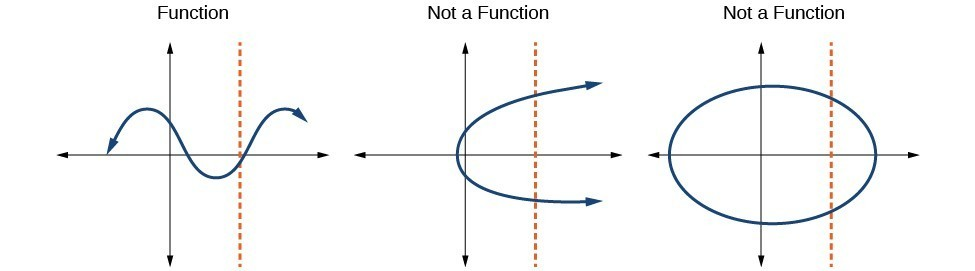Figure 12

### How To: Given a graph, use the vertical line test to determine if the graph represents a function.

1. Inspect the graph to see if any vertical line drawn would intersect the curve more than once.
2. If there is any such line, then the graph does not represent a function.

### Example 14: Applying the Vertical Line Test

Which of the graphs represent(s) a function $y=f\left(x\right)?$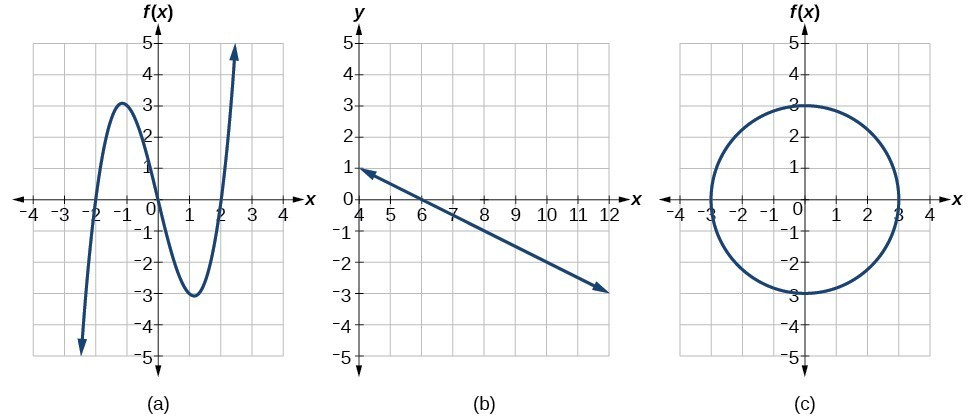Figure 13

### Try It

Does the graph in Figure 15 represent a function?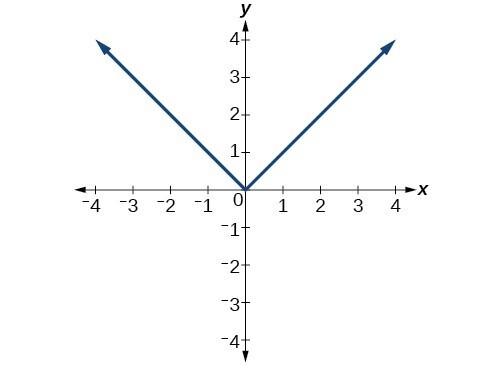Figure 15

# Identifying Basic Toolkit Functions

In this text we will be exploring functions—the shapes of their graphs, their unique characteristics, their algebraic formulas, and how to solve problems with them. When learning to read, we start with the alphabet. When learning to do arithmetic, we start with numbers. When working with functions, it is similarly helpful to have a base set of building-block elements. We call these our “toolkit functions,” which form a set of basic named functions for which we know the graph, formula, and special properties. Some of these functions are programmed to individual buttons on many calculators. For these definitions we will use $x$ as the input variable and $y=f\left(x\right)$ as the output variable.

We will see these toolkit functions, combinations of toolkit functions, their graphs, and their transformations frequently throughout this book. It will be very helpful if we can recognize these toolkit functions and their features quickly by name, formula, graph, and basic table properties. The graphs and sample table values are included with each function shown below.

Toolkit Functions
Name Function Graph
Constant $f\left(x\right)=c$, where $c$ is a constant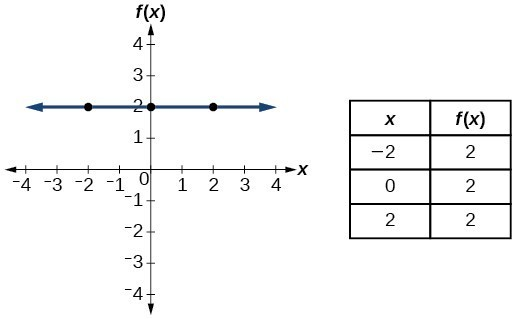Identity $f\left(x\right)=x$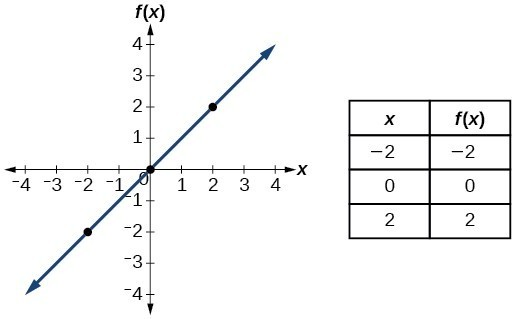Absolute value $f\left(x\right)=|x|$Quadratic $f\left(x\right)={x}^{2}$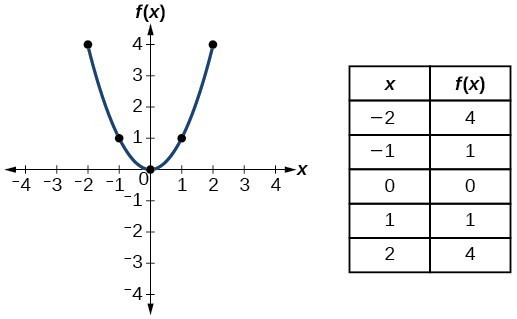Cubic $f\left(x\right)={x}^{3}$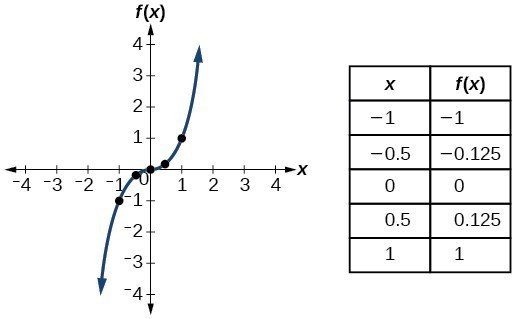Reciprocal $f\left(x\right)=\frac{1}{x}$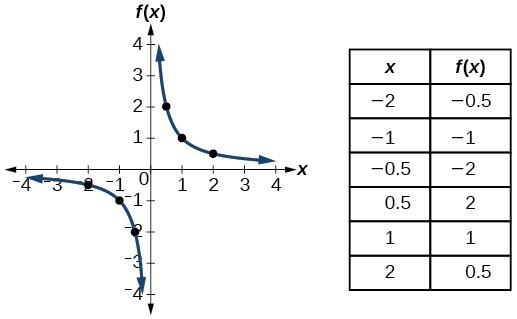Reciprocal squared $f\left(x\right)=\frac{1}{{x}^{2}}$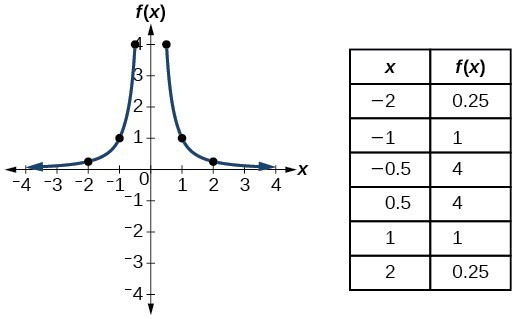Square root $f\left(x\right)=\sqrt{x}$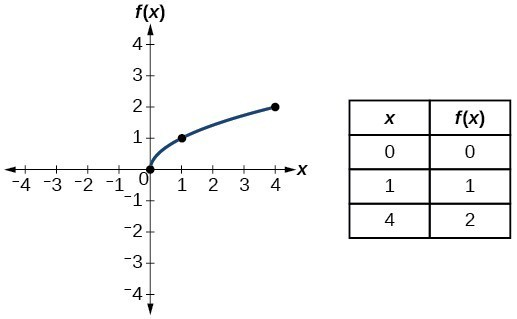Cube root $f\left(x\right)=\sqrt{x}$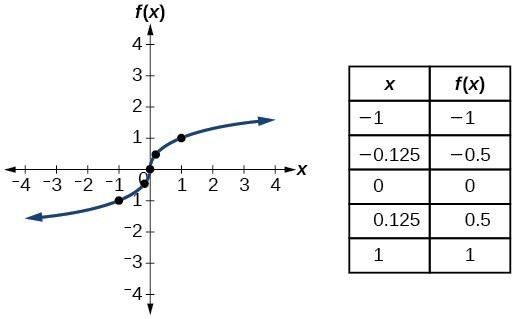## Key Equations

 Constant function $f\left(x\right)=c$, where $c$ is a constant Identity function $f\left(x\right)=x$ Absolute value function $f\left(x\right)=|x|$ Quadratic function $f\left(x\right)={x}^{2}$ Cubic function $f\left(x\right)={x}^{3}$ Reciprocal function $f\left(x\right)=\frac{1}{x}$ Reciprocal squared function $f\left(x\right)=\frac{1}{{x}^{2}}$ Square root function $f\left(x\right)=\sqrt{x}$ Cube root function $f\left(x\right)=\sqrt{x}$

## Key Concepts

• A relation is a set of ordered pairs. A function is a specific type of relation in which each domain value, or input, leads to exactly one range value, or output.
• Function notation is a shorthand method for relating the input to the output in the form $y=f\left(x\right)$.
• In tabular form, a function can be represented by rows or columns that relate to input and output values.
• To evaluate a function, we determine an output value for a corresponding input value. Algebraic forms of a function can be evaluated by replacing the input variable with a given value.
• To solve for a specific function value, we determine the input values that yield the specific output value.
• An algebraic form of a function can be written from an equation.
• Input and output values of a function can be identified from a table.
• Relating input values to output values on a graph is another way to evaluate a function.
• A graph represents a function if any vertical line drawn on the graph intersects the graph at no more than one point.

## Glossary

dependent variable
an output variable
domain
the set of all possible input values for a relation
function
a relation in which each input value yields a unique output value
independent variable
an input variable
input
each object or value in a domain that relates to another object or value by a relationship known as a function
output
each object or value in the range that is produced when an input value is entered into a function
range
the set of output values that result from the input values in a relation
relation
a set of ordered pairs
vertical line test
a method of testing whether a graph represents a function by determining whether a vertical line intersects the graph no more than once

## Section 1.3 Homework Exercises

1. What is the difference between a relation and a function?

2. What is the difference between the input and the output of a function?

3. Why does the vertical line test tell us whether the graph of a relation represents a function?

For the following exercises, determine whether the relation represents a function.

4. $\{(a,b),(c,d),(a,c)\}$

5. $\{(a,b),(b,c),(c,c)\}$

For the following exercises, determine whether the relation represents $y$ as a function of $x$.

6. $5x+2y=10$

7. $y=x^{2}$

8. $x=y^{2}$

9. $3x^{2}+y=14$

10. $2x+y^{2}=6$

11. $y=−2x^{2}+40x$

12. $y=\frac{1}{x}$

13. $x=\frac{3y+5}{7y−1}$

14. $x=\sqrt{1−y^2}$

15. $y=\frac{3x+5}{7x−1}$

16. $x^2+y^2=9$

17. $2xy=1$

18. $x=y^3$

19. $y=x^3$

20. $y=\sqrt{1−x^2}$

21. $x=\pm\sqrt{1−y}$

22. $y=\pm\sqrt{1−x}$

23. $y^2=x^2$

24. $y^3=x^2$

For the following exercises, evaluate the function $f$ at the indicated values $f(−3),f(2),f(−a),−f(a),f(a+h)$.

25. $f(x)=2x−5$

26. $f(x)=−5x^2+2x−1$

27. $f(x)=\sqrt{2−x}+5$

28. $f(x)=\frac{6x−15}{x+2}$

29. $f(x)=|x−1|−|x+1|$

30. Given the function $g(x)=5−x^{2}$,evaluate $g(x+h)−g(x)h,h\ne{0}$.

31. Given the function $g(x)=x^{2}+2x$,evaluate $g(x)−g(a)x−a,x\ne{a}$.

32. Given the function $k(t)=2t−1$:

Evaluate $k(2)$.

Solve $k(t)=7$.

33. Given the function $f(x)=8−3x$:
Evaluate $f(−2)$.
Solve $f(x)=−1$.

34. Given the function $p(c)=c^2+c$:
Evaluate $p(−3)$.
Solve $p(c)=2$.

35. Given the function $f(x)=x^2−3x$:
Evaluate $f(5)$.
Solve $f(x)=4$.

36. Given the function $f(x)=\sqrt{x+2}$:
Evaluate $f(7)$.
Solve $f(x)=4$.

37. Consider the relationship $3r+2t=18$.
Write the relationship as a function $r=f(t)$.
Evaluate $f(−3)$.
Solve $f(t)=2$.

For the following exercises, use the vertical line test to determine which graphs show relations that are functions.

38.39.40.41.42.43.44.45.46.47.48.49.50. Given the following graph,
Evaluate $f(−1)$.
Solve for $f(x)=3$.51. Given the following graph,
Evaluate $f(0$).
Solve for $f(x)=−3$.52. Given the following graph,
Evaluate $f(4)$.
Solve for $f(x)=1$.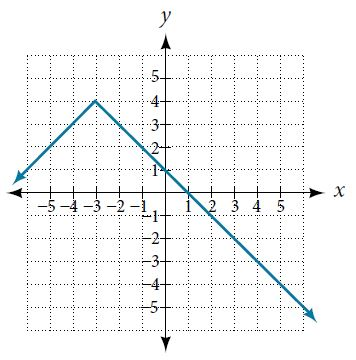For the following exercises, determine whether the relation represents a function.

53. $\{(−1,−1),(−2,−2),(−3,−3)\}$

54. $\{(3,4),(4,5),(5,6)\}$

55. $\{(2,5),(7,11),(15,8),(7,9)\}$

For the following exercises, determine if the relation represented in table form represents $y$ as a function of $x$.

56.

 x 5 10 15 y 3 8 14

57.

 x 5 10 15 y 3 8 8

58.
For the following exercises, use the function $f$ represented in the table below.

 $x$ $f$ 0 74 1 28 2 1 3 53 4 56 5 3 6 36 7 45 8 14 9 47

59. Evaluate $f(3)$.

60. Solve $f(x)=1$.

For the following exercises, evaluate the function $f$ at the values $f(−2),f(−1),f(0),f(1),\text{ and }f(2)$.

61. $f(x)=4−2x$

62. $f(x)=8−3x$

63. $f(x)=8x^2−7x+3$

64. $f(x)=3+\sqrt{x+3}$

65. $f(x)=\frac{x-2}{x+3}$

66. $3^x$

For the following exercises, evaluate the expressions, given functions $f,g,\text{ and }h$:

$f(x)=3x−2$
$g(x)=5−x^2$
$h(x)=−2x^2+3x−1$

67. $3f(1)−4g(−2)$

68. $f(73)−h(−2)$

69. The amount of garbage, $G$, produced by a city with population $p$ is given by $G=f(p)$. $G$ is measured in tons per week, and $p$ is measured in thousands of people. The town of Tola has a population of 40,000 and produces 13 tons of garbage each week. Express this information in terms of the function $f$. Explain the meaning of the statement $f(5)=2$.

70. The number of cubic yards of dirt, $D$,needed to cover a garden with area a square feet is given by $D=g(a)$. A garden with area 5000 ft2 requires 50 yd<sup>3</sup> of dirt. Express this information in terms of the function $g$.  Explain the meaning of the statement $g(100)=1$.

71. Let $f(t)$ be the number of ducks in a lake $t$ years after 1990. Explain the meaning of each statement:
$f(5)=30$
$f(10)=40$

72. Let $h(t)$ be the height above ground, in feet, of a rocket $t$ seconds after launching. Explain the meaning of each statement:
$h(1)=200$
$h(2)=350$

73. Show that the function $f(x)=3(x−5)^2+7$ is not one-to-one.